Resources tagged with: Area - triangles, quadrilaterals, compound shapes

Filter by: Content type:
Age range:
Challenge level:

There are 43 results

Broad Topics > Measuring and calculating with units > Area - triangles, quadrilaterals, compound shapes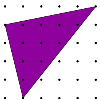Triangles in a Square

Age 11 to 14Challenge Level

What are the possible areas of triangles drawn in a square?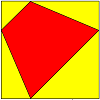Age 11 to 14Challenge Level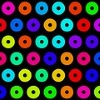Isometric Areas

Age 11 to 14Challenge Level

We usually use squares to measure area, but what if we use triangles instead?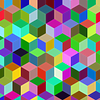More Isometric Areas

Age 11 to 14Challenge Level

Isometric Areas explored areas of parallelograms in triangular units. Here we explore areas of triangles...Triangle Transformation

Age 7 to 14Challenge Level

Start with a triangle. Can you cut it up to make a rectangle?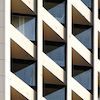Age 11 to 14Challenge Level

We started drawing some quadrilaterals - can you complete them?Dividing the Field

Age 14 to 16Challenge Level

A farmer has a field which is the shape of a trapezium as illustrated below. To increase his profits he wishes to grow two different crops. To do this he would like to divide the field into two. . . .Kissing Triangles

Age 11 to 14Challenge Level

Determine the total shaded area of the 'kissing triangles'.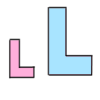Growing Rectangles

Age 11 to 14Challenge Level

What happens to the area and volume of 2D and 3D shapes when you enlarge them?Inscribed in a Circle

Age 14 to 16Challenge Level

The area of a square inscribed in a circle with a unit radius is, satisfyingly, 2. What is the area of a regular hexagon inscribed in a circle with a unit radius?Trapezium Four

Age 14 to 16Challenge Level

The diagonals of a trapezium divide it into four parts. Can you create a trapezium where three of those parts are equal in area?Biggest Enclosure

Age 14 to 16Challenge Level

Three fences of different lengths form three sides of an enclosure. What arrangement maximises the area?Six Discs

Age 14 to 16Challenge Level

Six circular discs are packed in different-shaped boxes so that the discs touch their neighbours and the sides of the box. Can you put the boxes in order according to the areas of their bases?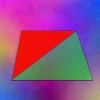Same Height

Age 14 to 16Challenge Level

A trapezium is divided into four triangles by its diagonals. Can you work out the area of the trapezium?Squ-areas

Age 14 to 16Challenge Level

Three squares are drawn on the sides of a triangle ABC. Their areas are respectively 18 000, 20 000 and 26 000 square centimetres. If the outer vertices of the squares are joined, three more. . . .Age 11 to 14Challenge Level

Four rods, two of length a and two of length b, are linked to form a kite. The linkage is moveable so that the angles change. What is the maximum area of the kite?At a Glance

Age 14 to 16Challenge Level

The area of a regular pentagon looks about twice as a big as the pentangle star drawn within it. Is it?From All Corners

Age 14 to 16Challenge Level

Straight lines are drawn from each corner of a square to the mid points of the opposite sides. Express the area of the octagon that is formed at the centre as a fraction of the area of the square.Isosceles

Age 11 to 14Challenge Level

Prove that a triangle with sides of length 5, 5 and 6 has the same area as a triangle with sides of length 5, 5 and 8. Find other pairs of non-congruent isosceles triangles which have equal areas.Dotty Triangles

Age 11 to 14Challenge Level

Imagine an infinitely large sheet of square dotty paper on which you can draw triangles of any size you wish (providing each vertex is on a dot). What areas is it/is it not possible to draw?Tilted Squares

Age 11 to 14Challenge Level

It's easy to work out the areas of most squares that we meet, but what if they were tilted?Rati-o

Age 11 to 14Challenge Level

Points P, Q, R and S each divide the sides AB, BC, CD and DA respectively in the ratio of 2 : 1. Join the points. What is the area of the parallelogram PQRS in relation to the original rectangle?Equilateral Areas

Age 14 to 16Challenge Level

ABC and DEF are equilateral triangles of side 3 and 4 respectively. Construct an equilateral triangle whose area is the sum of the area of ABC and DEF.Rhombus in Rectangle

Age 14 to 16Challenge Level

Take any rectangle ABCD such that AB > BC. The point P is on AB and Q is on CD. Show that there is exactly one position of P and Q such that APCQ is a rhombus.Age 14 to 16Challenge Level

In this problem we are faced with an apparently easy area problem, but it has gone horribly wrong! What happened?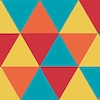Triangle in a Trapezium

Age 11 to 16Challenge Level

Can you find and prove the relationship between the area of a trapezium and the area of a triangle constructed within it?Age 14 to 16Challenge Level

Investigate the properties of quadrilaterals which can be drawn with a circle just touching each side and another circle just touching each vertex.Towering Trapeziums

Age 14 to 16Challenge Level

Can you find the areas of the trapezia in this sequence?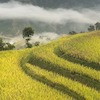The Farmers' Field Boundary

Age 11 to 14Challenge Level

The farmers want to redraw their field boundary but keep the area the same. Can you advise them?Isosceles Triangles

Age 11 to 14Challenge Level

Draw some isosceles triangles with an area of $9$cm$^2$ and a vertex at (20,20). If all the vertices must have whole number coordinates, how many is it possible to draw?Age 14 to 16Challenge Level

The points P, Q, R and S are the midpoints of the edges of a non-convex quadrilateral.What do you notice about the quadrilateral PQRS and its area?Areas of Parallelograms

Age 14 to 16Challenge Level

Can you find the area of a parallelogram defined by two vectors?Shear Magic

Age 11 to 14Challenge Level

Explore the area of families of parallelograms and triangles. Can you find rules to work out the areas?Of All the Areas

Age 14 to 16Challenge Level

Can you find a general rule for finding the areas of equilateral triangles drawn on an isometric grid?Age 14 to 16Challenge LevelDisappearing Square

Age 11 to 14Challenge Level

Do you know how to find the area of a triangle? You can count the squares. What happens if we turn the triangle on end? Press the button and see. Try counting the number of units in the triangle now. . . .Gutter

Age 14 to 16Challenge Level

Manufacturers need to minimise the amount of material used to make their product. What is the best cross-section for a gutter?Pick's Theorem

Age 14 to 16Challenge Level

Polygons drawn on square dotty paper have dots on their perimeter (p) and often internal (i) ones as well. Find a relationship between p, i and the area of the polygons.Kite in a Square

Age 14 to 16Challenge Level

Can you make sense of the three methods to work out the area of the kite in the square?Square Pizza

Age 14 to 16Challenge Level

Can you show that you can share a square pizza equally between two people by cutting it four times using vertical, horizontal and diagonal cuts through any point inside the square?Overlap

Age 11 to 14Challenge Level

A red square and a blue square overlap so that the corner of the red square rests on the centre of the blue square. Show that, whatever the orientation of the red square, it covers a quarter of the. . . .Maths Filler 2

Age 14 to 16Challenge Level

Can you draw the height-time chart as this complicated vessel fills with water?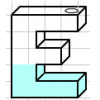Maths Filler

Age 11 to 14Challenge Level

Imagine different shaped vessels being filled. Can you work out what the graphs of the water level should look like?